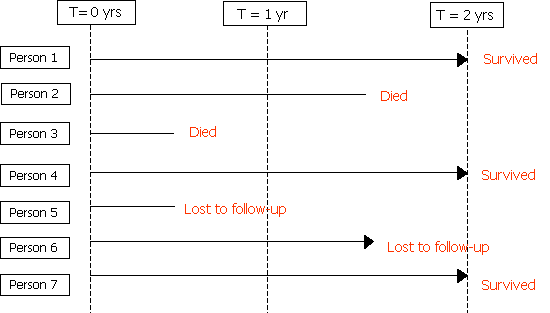# Risk and hazard

## Risk and hazard

Introduction

Risk and hazard are terms commonly used to describe aspects of the potential for harm from environmental and other agents.  The meaning of these terms in epidemiological usage is somewhat different from their meaning in common parlance, and indeed different from their usage in disciplines concerned with risk management.  In this section, the epidemiological definitions are presented which consider risk as a probability (without reference to the potential size of the adverse health impact), and hazard as simply an agent with potential to cause harm.  The distinction with risk management terminology is explained, as well as other terms relating to exposures and measures of disease occurrence.  Yet another variant of the definition of hazard is given in section 8 (health and safety).

Key definitions and terms

 Exposure The (degree of) contact with a hazardous agent of disease such that harmful effects or disease transmission may occur. Commonly used to refer a measure of the amount of a hazardous agent to which an individual is exposed. Hazard A factor or exposure that may adversely affect health; anything that has the potential to cause harm. Hazard rate A theoretical measure of the risk (probability) of occurrence of an event, e.g. death or new disease, at a point in time, t. It is defined mathematically as the limit, as dt approaches zero, of the probability that an individual at time t will experience the event by t + dt, divided by dt.  This is sometimes referred to simply as 'the hazard', hence 'hazard function'. Rate The number of new disease cases occurring among a defined population calculated per person-time of follow-up. Risk (1) Epidemiological definition The probability that an event will occur e.g. that an individual will become ill or die within a stated period of time or age.  Formally defined as the proportion of initially disease free individuals who develop disease over a defined period of observation. (2) Risk management definition The probability that an adverse event will occur (such as exposure from a chemical incident) times the consequences of the adverse event Relative risk / risk ratio The ratio of two risks (or, informally, of rates or odds) comparing the risk of disease or death among the exposed to the risk among the unexposed.

Risk and hazard

Risk

Risk has both technical and common-usage definitions.  In everyday usage it refers to 'the chance of danger, loss, injury or other adverse consequence' (Oxford English Dictionary) – a risk to health etc.  Sometimes it is taken to mean an indicator of threat or the combination of probability and impact, e.g.

 risk = probability of an accident X losses per accident

Thus similar risk may be obtained by high impact but low probability or by low impact and high probability of occurring.  By this definition, risks are assessed by rating their impact and their probability to yield a product risk score.

Epidemiological definition of risk

However, in epidemiology the definition of risk is purely one of probability or chance, as measured by the occurrence of new cases of disease in a defined population over a defined period:

 risk = number of (new) observed cases number at risk (disease free) at the start

This is the definition favoured by the US Environmental Protection Agency.

Rate

Risk is distinguished from disease rate, which by convention is defined in terms of person-time of follow-up:

 rate = number of observed cases = d person time (years) at risk ∑ti

where ti is the period of observation (time at risk, follow-up time) of the ith individual.

Rates are calculable in circumstances when the time at risk is known, such as in the follow-up of cohorts.

A rate therefore has units (cases per person per year), whereas risk does not, as it is a simple probability or proportion.  The distinction between a risk and a rate is shown diagrammatically below.There were two deaths, so d=2.  The total person time of follow-up is 2 years for individuals 1, 4 and 7; one-and-a-half years for persons 2 and 6 (person 6 was lost to follow-up after one-and-a-half years); and half a year for persons 3 and 5 (person 5 was lost to follow-up after half a year).  Thus:

 Risk = d / N = 2 / 7 = 0.29 Rate = d / ∑ti = 2 / (2 + 1.5 + 0.5 + 2 + 0.5 + 1.5 + 2) = 2/10 = 0.2  deaths/person-year

Risk perception

Perception of risk – i.e. the subjective judgment that people make about the characteristics and severity of a risk – is often important for environmental exposures, such as air pollution or nuclear power.  Rankings of hazards based on perception are often not the same as those based on objective probabilities. Several factors appear to influence the way people interpret environmental risks, among them are:

• the degree to which the risk is perceived as controllable or exposure as avoidable
• the level of knowledge/certainty about its nature and effects
• the trustworthiness of the authorities that provide information about it and are responsible for managing it.

Nuclear power, for example, is often looked upon with particular fear because it is poorly understood, the level of risks are uncertain, government authorities responsible for managing it are often not trusted.

Hazard

In its strict sense, a hazard is simply something which could potentially be harmful to a person's life or well-being.  However, hazards are sometimes classified by the combination of the likelihood of the hazard turning into a (health) effect and by the seriousness of that effect (see also the discussion of risk above).  This type of consideration tends to indicate protective action when the probability of an adverse effect is high or its impact is high (even if the probability is low) or both.

Useful websites

© Dr Paul Wilkinson 2009, Rebecca Close and Helen Crabbe 2016